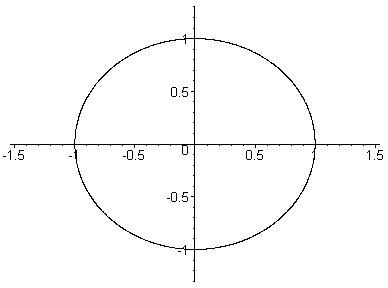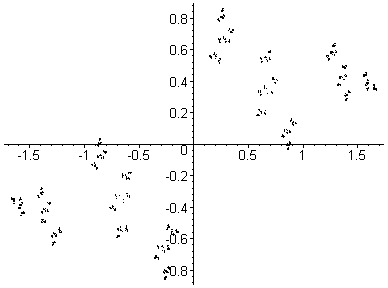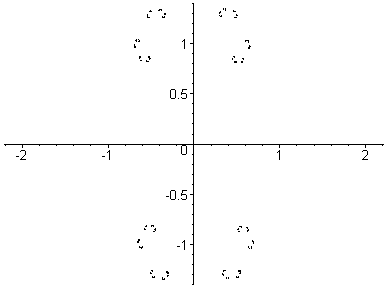## Julia Sets

This page has animated gif files illustrating the changing shapes of Julia sets as the parameter c changes. For some examples of 'static' Julia sets, see the Julia page.

See also the Julia Sets Movie applet on the software page.

The gif files on this page are about 160kb each so it may take a while to download them.

Julia sets are parameterized by a complex number c = (a,b). For some values of c the Julia set is one piece ('connected'), while for other values of c the Julia set is dust ('totally disconnected'). The Mandelbrot set classifies the complex numbers into two groups; those c's where the Julia set is connected, and those c's where the Julia set is disconnected (every complex number is in exactly one of the two groups). As c varies the shape of the Julia set varies. The animated gif files here show you several scenarios of Julia sets as c changes. See if you can tell when the Julia set breaks apart inot dust (if it does).

See the W00 course notes on Julia sets for more information.

These animated gif files were produced with MAPLE6. You may download the program here , or view the code (in plain text) here .A Julia set breaking apart into dust. Here, c goes from (0,0) to (0,1.6) in 26 steps. This is a path up through the main body of the Mandelbrot set past the Misiurewicz point (0,1). For c=(0,0) the Julia set is a circle (of radius 1). For c=(0,1.6) the Julia set is dust. At c=(0,1) the Julia set is a 'line'. Each Julia set drawn here contains 32,768 points.Here, c goes from (-1,-0.8) to (-1,0.8) in 26 steps. This is a path up through the 'neck' of the Mandelbrot set. Note that the point where he 'head' joins the 'body' of the Mandelbrot set is a parabolic point; the associated Julia set is just about to break apart. Each Julia set drawn here contains 32,768 points.Here, c goes from (1,0.05) to (-2.4,0.05) in 29 steps. This is a path from right to left through the centre of the Mandelbrot set. Each Julia set drawn here contains 2,048 points.

More animated Julia sets.